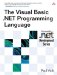# Statements

 <  Day Day Up  >

 There are several kinds of statements. Assignment statements ” In addition to the standard assignment statement ( = ), compound assignment operators use the left-hand side of the assignment as the left operand of the operator. The following example adds 10 to the value 5 and then divides the result by 3. ` Dim x As Integer = 5 x += 10 x \= 3 ` Looping statements ” There are four kinds of loop statements: While statements, Do statements, For statements, and For Each statements. While and Do statements repeat a set of statements based on a condition. ` Dim x As Integer While x < 5 x += 1 End While Do x -= 1 Loop While x > 0 ` For statements increment a variable from one bound to another bound. A step value can be specified that indicates how much the variable should be incremented on each loop, and the step value can be negative. The following example loops from zero to ten, stepping by two each time. ` For x As Integer = 0 To 10 Step 2 Console.WriteLine(x) Next x ` For Each statements loop over all the items in an array or a list of values. ` Dim c() As Integer = New Integer() { 1, 2, 3 } For Each x As Integer In c Console.WriteLine(x) Next x ` With statement ” The With statement simplifies accessing multiple members of a value by allowing the repeated expression to be omitted. So the following code: ` Dim c As ArrayList = New ArrayList() c.Add(1) c.Add(2) c.Add(3) ` can instead be written as follows : ` Dim c As ArrayList = New ArrayList() With c .Add(1) .Add(2) .Add(3) End With ` Conditional statements ” The If conditional statement executes code based on the value of a Boolean expression. ` Dim x As Integer If x < 0 Then Console.WriteLine("Less than zero.") Else Console.WriteLine("Not less than zero.") End If ` The Select statement executes code based on a series of conditions. ` Dim x As String Select Case x Case "Red" Console.WriteLine("Red") Case "Green" Console.WriteLine("Green") Case "Blue" Console.WriteLine("Blue") End Select ` Branching statements ” Most block statements have a corresponding Exit statement that immediately exits the block. ` Dim x As Integer While True x = CInt(Console.ReadLine()) If x = 0 Then Exit While End If End While ` Visual Basic .NET also supports the GoTo statement, which branches to a label. ` Dim x As Integer While True x = CInt(Console.ReadLine()) If x = 0 Then GoTo ExitLoop End If End While ExitLoop: Console.WriteLine("Finished.") ` The Return statement exits a subroutine or function. Return can also specify the return value of a function. ` Function Add(ByVal x As Integer, ByVal y As Integer) As Integer Return x + y End Function ` The Stop statement causes the application to stop executing and break into the debugger, if one is available. The End statement causes the application to stop executing entirely. SyncLock statement ” The SyncLock statement can be used to prevent more than one thread of execution from executing the same block of code. The argument to the statement is a value that can be used by the Framework as a lock. The following example uses two threads of execution to fill up an array with 10,000 values. A lock is used to prevent the threads from writing to the same location in the array. ` Imports System.Threading Module Test Dim Values(10000) As Integer Dim CurrentIndex As Integer = 0 Sub FillValues() For Number As Integer = 1 to 5000 SyncLock Values.SyncRoot Values(CurrentIndex) = Number CurrentIndex += 1 End SyncLock Next Number End Sub Sub Main() Dim t1 As Thread = New Thread(AddressOf FillValues) Dim t2 As Thread = New Thread(AddressOf FillValues) t1.Start() t2.Start() End Sub End Module `
 <  Day Day Up  >The Visual Basic .NET Programming Language
ISBN: 0321169514
EAN: 2147483647
Year: 2004
Pages: 173
Authors: Paul Vick

Similar book on Amazon